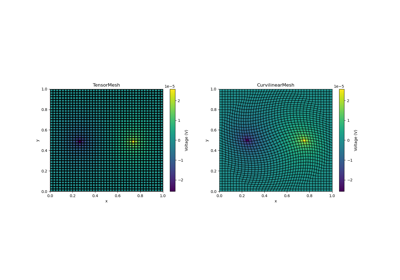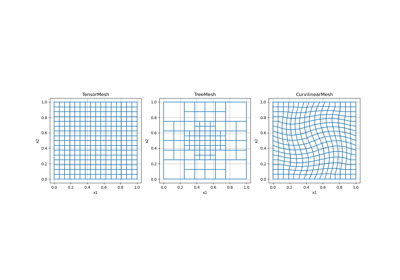# discretize.CurvilinearMesh#class discretize.CurvilinearMesh(node_list, **kwargs)[source]#

Curvilinear mesh class.

Curvilinear meshes are numerical grids whose cells are general quadrilaterals (2D) or cuboid (3D); unlike tensor meshes (see `TensorMesh`) whose cells are rectangles or rectangular prisms. That being said, the combinatorial structure (i.e. connectivity of mesh cells) of curvilinear meshes is the same as tensor meshes.

Parameters:
node_list`list` `of` array_like

List `array_like` containing the gridded x, y (and z) node locations.

• For a 2D curvilinear mesh, node_list = [X, Y] where X and Y have shape (`n_nodes_x`, `n_nodes_y`)

• For a 3D curvilinear mesh, node_list = [X, Y, Z] where X, Y and Z have shape (`n_nodes_x`, `n_nodes_y`, `n_nodes_z`)

Examples

Using the `example_curvilinear_grid()` utility, we provide an example of a curvilinear mesh.

```>>> from discretize import CurvilinearMesh
>>> from discretize.utils import example_curvilinear_grid
>>> import matplotlib.pyplot as plt
```

The example grid slightly rotates the nodes in the center of the mesh,

```>>> x, y = example_curvilinear_grid([10, 10], "rotate")
>>> x.shape
(11, 11)
>>> y.shape
(11, 11)
>>> curvilinear_mesh = CurvilinearMesh([x, y])
>>> curvilinear_mesh.shape_nodes
(11, 11)
```
```>>> fig = plt.figure(figsize=(5,5))
>>> curvilinear_mesh.plot_grid(ax=ax)
>>> plt.show()
```

Attributes

 `area` area has been deprecated. `average_cell_to_edge` Averaging operator from cell centers to edges (scalar quantities). `average_cell_to_face` Averaging operator from cell centers to faces (scalar quantities). `average_cell_vector_to_face` Averaging operator from cell centers to faces (vector quantities). `average_edge_to_cell` Averaging operator from edges to cell centers (scalar quantities). `average_edge_to_cell_vector` Averaging operator from edges to cell centers (vector quantities). `average_edge_to_face` Averaging operator from edges to faces. `average_edge_x_to_cell` Averaging operator from x-edges to cell centers (scalar quantities). `average_edge_y_to_cell` Averaging operator from y-edges to cell centers (scalar quantities). `average_edge_z_to_cell` Averaging operator from z-edges to cell centers (scalar quantities). `average_face_to_cell` Averaging operator from faces to cell centers (scalar quantities). `average_face_to_cell_vector` Averaging operator from faces to cell centers (vector quantities). `average_face_x_to_cell` Averaging operator from x-faces to cell centers (scalar quantities). `average_face_y_to_cell` Averaging operator from y-faces to cell centers (scalar quantities). `average_face_z_to_cell` Averaging operator from z-faces to cell centers (scalar quantities). `average_node_to_cell` Averaging operator from nodes to cell centers (scalar quantities). `average_node_to_edge` Averaging operator from nodes to edges (scalar quantities). `average_node_to_face` Averaging operator from nodes to faces (scalar quantities). `axis_u` Orientation of the first axis. `axis_v` Orientation of the second axis. `axis_w` Orientation of the third axis. `boundary_edge_vector_integral` Integrate a vector function on the boundary. `boundary_edges` Boundary edge locations. `boundary_face_outward_normals` Outward normal vectors of boundary faces. `boundary_face_scalar_integral` Represent the operation of integrating a scalar function on the boundary. `boundary_faces` Boundary face locations. `boundary_node_vector_integral` Integrate a vector function dotted with the boundary normal. `boundary_nodes` Boundary node locations. `cellGrad` cellGrad has been deprecated. `cellGradBC` cellGradBC has been deprecated. `cellGradx` cellGradx has been deprecated. `cellGrady` cellGrady has been deprecated. `cellGradz` cellGradz has been deprecated. `cell_centers` Return gridded cell center locations. `cell_gradient` Cell gradient operator (cell centers to faces). `cell_gradient_BC` Boundary conditions matrix for the cell gradient operator (Deprecated). `cell_gradient_x` X-derivative operator (cell centers to x-faces). `cell_gradient_y` Y-derivative operator (cell centers to y-faces). `cell_gradient_z` Z-derivative operator (cell centers to z-faces). `cell_volumes` Return cell volumes. `dim` The dimension of the mesh (1, 2, or 3). `edge` edge has been deprecated. `edgeCurl` edgeCurl has been deprecated. `edge_curl` Edge curl operator (edges to faces). `edge_lengths` Return lengths of all edges in the mesh. `edge_tangents` Unit tangent vectors for all mesh edges. `edges` Gridded edge locations. `edges_x` Gridded x-edge locations (staggered grid). `edges_y` Gridded y-edge locations (staggered grid). `edges_z` Gridded z-edge locations (staggered grid). `faceDiv` faceDiv has been deprecated. `faceDivx` faceDivx has been deprecated. `faceDivy` faceDivy has been deprecated. `faceDivz` faceDivz has been deprecated. `face_areas` Return areas of all faces in the mesh. `face_divergence` Face divergence operator (faces to cell-centres). `face_normals` Unit normal vectors for all mesh faces. `face_x_divergence` X-derivative operator (x-faces to cell-centres). `face_y_divergence` Y-derivative operator (y-faces to cell-centres). `face_z_divergence` Z-derivative operator (z-faces to cell-centres). `faces` Gridded face locations. `faces_x` Gridded x-face locations (staggered grid). `faces_y` Gridded y-face locations (staggered grid). `faces_z` Gridded z-face locations (staggered grid). `nCx` Number of cells in the x direction. `nCy` Number of cells in the y direction. `nCz` Number of cells in the z direction. `nNx` Number of nodes in the x-direction. `nNy` Number of nodes in the y-direction. `nNz` Number of nodes in the z-direction. `n_cells` Total number of cells in the mesh. `n_edges` Total number of edges in the mesh. `n_edges_per_direction` The number of edges in each direction. `n_edges_x` Number of x-edges in the mesh. `n_edges_y` Number of y-edges in the mesh. `n_edges_z` Number of z-edges in the mesh. `n_faces` Total number of faces in the mesh. `n_faces_per_direction` The number of faces in each axis direction. `n_faces_x` Number of x-faces in the mesh. `n_faces_y` Number of y-faces in the mesh. `n_faces_z` Number of z-faces in the mesh. `n_nodes` Total number of nodes in the mesh. `nodalGrad` nodalGrad has been deprecated. `nodalLaplacian` nodalLaplacian has been deprecated. `nodal_gradient` Nodal gradient operator (nodes to edges). `nodal_laplacian` Nodal scalar Laplacian operator (nodes to nodes). `node_list` The gridded x, y (and z) node locations used to create the mesh. `nodes` Return gridded node locations. `normals` normals has been deprecated. `orientation` Rotation matrix defining mesh axes relative to Cartesian. `origin` Origin or 'anchor point' of the mesh. `project_edge_to_boundary_edge` Projection matrix from all edges to boundary edges. `project_face_to_boundary_face` Projection matrix from all faces to boundary faces. `project_node_to_boundary_node` Projection matrix from all nodes to boundary nodes. `reference_is_rotated` Indicate whether mesh uses standard coordinate axes. `reference_system` Coordinate reference system. `rotation_matrix` Alias for `orientation`. `shape_cells` Number of cells in each coordinate direction. `shape_edges_x` Number of x-edges along each axis direction. `shape_edges_y` Number of y-edges along each axis direction. `shape_edges_z` Number of z-edges along each axis direction. `shape_faces_x` Number of x-faces along each axis direction. `shape_faces_y` Number of y-faces along each axis direction. `shape_faces_z` Number of z-faces along each axis direction. `shape_nodes` The number of nodes along each axis direction. `stencil_cell_gradient` Stencil for cell gradient operator (cell centers to faces). `stencil_cell_gradient_x` Differencing operator along x-direction (cell centers to x-faces). `stencil_cell_gradient_y` Differencing operator along y-direction (cell centers to y-faces). `stencil_cell_gradient_z` Differencing operator along z-direction (cell centers to z-faces). `tangents` tangents has been deprecated. `vol` vol has been deprecated. `x0` Alias for the `origin`.

Methods

 `cell_gradient_weak_form_robin`([alpha, beta, ...]) Create Robin conditions pieces for weak form of the cell gradient operator (cell centers to faces). `closest_points_index`(locations[, grid_loc, ...]) Find the indicies for the nearest grid location for a set of points. Make a copy of the current mesh. `deserialize`(value, **kwargs) Create this mesh from a dictionary of attributes. `edge_divergence_weak_form_robin`([alpha, ...]) Create Robin conditions pieces for weak form of the edge divergence operator (edges to nodes). `equals`(other_mesh) Compare the current mesh with another mesh to determine if they are identical. `from_omf`(element) Convert an `omf` object to a `discretize` mesh. `getBCProjWF`(*args, **kwargs) getBCProjWF has been removed. `getBCProjWF_simple`(*args, **kwargs) getBCProjWF_simple has been removed. `getEdgeInnerProduct`(*args, **kwargs) getEdgeInnerProduct has been removed. `getEdgeInnerProductDeriv`(*args, **kwargs) getEdgeInnerProductDeriv has been removed. `getFaceInnerProduct`(*args, **kwargs) getFaceInnerProduct has been removed. `getFaceInnerProductDeriv`(*args, **kwargs) getFaceInnerProductDeriv has been removed. `getInterpolationMat`(*args, **kwargs) getInterpolationMat has been removed. `get_BC_projections`(BC[, discretization]) Create the weak form boundary condition projection matrices. `get_BC_projections_simple`([discretization]) Create weak form boundary condition projection matrices for mixed boundary condition. `get_edge_inner_product`([model, ...]) Generate the edge inner product matrix or its inverse. `get_edge_inner_product_deriv`(model[, ...]) Get a function handle to multiply vector with derivative of edge inner product matrix (or its inverse). `get_face_inner_product`([model, ...]) Generate the face inner product matrix or its inverse. `get_face_inner_product_deriv`(model[, ...]) Get a function handle to multiply a vector with derivative of face inner product matrix (or its inverse). `get_interpolation_matrix`(loc[, ...]) Construct a linear interpolation matrix from mesh. `plotGrid`(*args, **kwargs) plotGrid has been deprecated. `plotImage`(*args, **kwargs) plotImage has been deprecated. `plotSlice`(*args, **kwargs) plotSlice has been deprecated. `plot_3d_slicer`(v[, xslice, yslice, zslice, ...]) Plot slices of a 3D volume, interactively (scroll wheel). `plot_grid`([ax, nodes, faces, centers, ...]) Plot the grid for nodal, cell-centered and staggered grids. `plot_image`(v[, v_type, grid, view, ax, ...]) Plot quantities defined on a given mesh. `plot_slice`(v[, v_type, normal, ind, ...]) Plot a slice of fields on the given 3D mesh. `point2index`(locs) Find cells that contain the given points. `projectEdgeVector`(*args, **kwargs) projectEdgeVector has been removed. `projectFaceVector`(*args, **kwargs) projectFaceVector has been removed. `project_edge_vector`(edge_vectors) Project vectors to the edges of the mesh. `project_face_vector`(face_vectors) Project vectors onto the faces of the mesh. `r`(*args, **kwargs) r has been removed. `reshape`(x[, x_type, out_type, return_format]) Reshape tensor quantities. `save`([file_name, verbose]) Save the mesh to json. Represent the mesh's attributes as a dictionary. `setCellGradBC`(*args, **kwargs) setCellGradBC has been removed. Set boundary conditions for derivative operators acting on cell-centered quantities. `toVTK`([models]) Convert mesh (and models) to corresponding VTK or PyVista data object. Represent the mesh's attributes as a dictionary. `to_omf`([models]) Convert to an `omf` data object. `to_vtk`([models]) Convert mesh (and models) to corresponding VTK or PyVista data object. Return the validation state of the mesh. `writeVTK`(file_name[, models, directory]) Convert mesh (and models) to corresponding VTK or PyVista data object then writes to file. `write_vtk`(file_name[, models, directory]) Convert mesh (and models) to corresponding VTK or PyVista data object then writes to file.

## Galleries and Tutorials using `discretize.CurvilinearMesh`#Basic Forward 2D DC Resistivity

Basic Forward 2D DC ResistivityOverview of Mesh Types

Overview of Mesh Types